# Coherence

June 2, 2021

There are two definitions of coherence in literature: complex and ordinary.

Complex:

(1)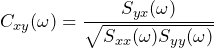Ordinary:

(2)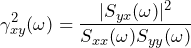Where:

• Sxx(f) is the PSD of x
• Syy(f) is the PSD of y
• Syx(f) is the CSD of x and y

ObserVIEW currently offers complex coherence. To calculate ordinary coherence, take the magnitude squared of the complex coherence.

#### Notes

Of the two definitions, ordinary coherence is more common in literature. However, ordinary coherence is the magnitude squared of complex coherence.

(3)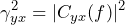Thus, ordinary coherence is a special case of the more general complex coherence.

### Applications

Ordinary coherence has two main practical uses.

1. Coherence is a measure of confidence that a peak observed in a transfer function is a resonant frequency of the device under test and not a spike due to measurement noise. For example, suppose that a peak at frequency f in a transfer function is observed. Then:
γyx2 |Cyx|

Description

γyx2 ≥ 0.70 or |Cyx|≥ 0.85

High degree of confidence

γyx2 ≤ 0.50 or |Cyx| ≤ 0.707

Not likely

0.50 < γyx2 < 0.70 or 0.707 < |Cyx| < 0.85

Somewhat likely

1. Coherence is a measure of linearity of the system under test at frequency f.

An ordinary coherence value near 1 indicates that the system is linear-time-invariant (LTI) at frequency f. An ordinary coherence value near 0 indicates that the system is non-linear, statistically changing with time or both.

Coherence drops within a resonance, and one must evaluate surrounding coherence values to make a sound judgment.

### Coherence as a Measure of Linearity

In many cases, the signal x may represent an input to a system and the signal y may represent the output. Suppose that an input to a system results in a response h[n] and that the DTFT of the response is H(ω). If the system is LTI, then Syx(ω) = H(ω) Sxx(ω) and Syy(ω) = |H(ω)|2 Sxx(ω).

Also, the coherence will be:

 (4)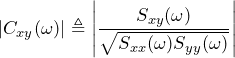by definition of coherence Cxy

(5)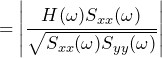(6)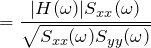(7)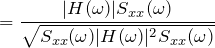(8)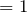Thus, for an LTI system, the ordinary coherence γyx2(f) will be 1 for all frequencies. In contrast, if the coherence is less than 1, then it is not LTI. Further, if γyx2(f) is 1 at certain frequencies, then the system is LTI at those frequencies.

### Coherence as a Measure of Confidence in Transfer Function H1

If a system is LTI with a frequency response of Syx(ω) = H(ω) Sxx(ω), then H(ω) = Syx(ω)/Sxx(ω), which is the transfer function H1. Thus, if one wants a measure of how good an estimator H1 is likely to be, they can check the ordinary coherence function γyx2(f).

If γyx2(f) is 1 for all or nearly all frequencies, then Hyx1(f) is likely a good Hyx1 estimator. If γyx2 is close to 0, then one should be much less confident about the quality of the Hyx1 estimator.

### Coherence as a Measure of Distance Between H1 and H2

You can also view the ordinary-coherence function γyx2(f) as a measure of the distance between Hyx1 and Hyx2.

(9)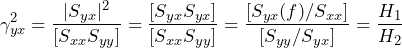As 0 ≤ γyx2(f) ≤ 1, then 0 ≤ |H1/H2| ≤ 1. Therefore, |H1(f)| ≤ |H2(f)|.

When the true H is LTI, then H1=H2—meaning the distance is 0— and γyx2=1. If H is not LTI, then the distance will be greater than 0 and γyx2<1.

In the above example, the distance between |H1(435)|=19 and |H2(435)|=607 is great. This is reflected in the following equation:

γyx2(435) = |Cyx(435)|2 = (0.007)2 =(0.0098)2 = (19/607)2 = (|H1|/|H2|)2

### Note of Caution

As the name implies, estimators yield estimates. In general, these estimates contain some error compared to the true value. It would be difficult to find a more striking example of this situation than the K=1 Welch estimate of coherence.

#### Welch Estimate of Coherence

Compare the rectangular window K=1 Welch estimate of coherence with the true coherence of uncorrelated white noise signals. If:

1. x[n] and y[n] are zero-mean white noise sequences
2. x[n] and y[n] are uncorrelated with each other
3. The estimate Ĉyx (ω) is the K=1 Welch estimate of x and y

Then, Ĉyx(ω)= 1.

Proof:

 (10)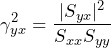by definition of ordinary coherence γyx(ω) (11)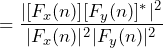by Welch algorithm

(12)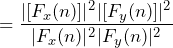(13)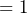Thus, the K=1 Welch estimate of coherence is always 1. It is an estimate, but is it a good estimate? To answer this question, we can compute the true (non-estimated) coherence.

 (14)by definition of ordinary coherence γyx(ω) (15)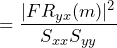by definition of CSD Syx(ω) (16)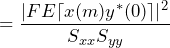by definition of cross-correlation Ryx(m) (17)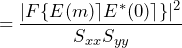x and y are uncorrelated (18)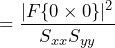x[n] and y[n] are zero-mean (19)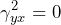because the Fourier transform F of 0 is 0

The true value of the coherence is γyx(ω)= 0, but the Welch estimate is γyx(ω)= 1. In many cases, Welch will likely yield a good estimate. However, as the above example demonstrates, this is not always the case, and some caution is necessary.